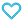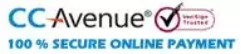# 44 + 20 Years Chapterwise Solutions Maths for JEE (Adv + Main)

625.00

Discount offer on this book in a bundle, click to view

MTG’s 44+20 years Chapterwise Solutions is a highly recommended book by toppers. This is the best book for students who wish to crack JEE Main and Advanced. It contains previous 44 years papers of JEE Advanced/ IIT JEE and 20 years papers of JEE Main/ AIEEE.  The book is provided with a chapter wise synopsis in the form of “Chapter in a Nutshell”. The synopsis helps the students in revising the chapters before starting the practice of questions.  Further, all the questions from JEE Main & Advanced are arranged in Chapter wise format.

849 in stock

Qty:Add to WishlistMTG’s 44+20 years chapterwise solution book is designed for those who are targeting JEE mains and advanced both. It contains previous 44 years papers of JEE advanced/IIT JEE and 20 years papers of JEE Main/AIEEE. The Book is divided into two sections, Class 11 and Class 12 as per the NCERT syllabus. Each chapter begins with synopsis (in a nutshell) to understand the concept and formulae for solving the problem in a better way.

Since JEE Main and Advanced questions are present in chapter wise format, it gives a better idea of difference in level of questions in two exams. It also guides students about the depth of understanding a topic required for a certain chapter and topic. The number of questions reflects which chapter is important for Mains and which is for Advanced. A student using this book strategically can come up with flying colors in both the exams.

Table of Content:
• CLASS XI
• 1. Sets
• 2. Trigonometric Functions
• 3. Properties of Triangles
• 4. Binomial Theorem and Mathematical Induction
• 5. Complex Numbers and Quadratic Equations
• 6. Permutations and Combinations
• 7. Sequences and Series
• 8. Straight Lines
• 9. Conic Sections
• 10. Limits and Derivatives
• 11. Mathematical Reasoning
• 12. Statistics
• 13. Logarithms
• CLASS XII
• 1. Relations and Functions
• 2. Inverse Trigonometric Functions
• 3. Matrices and Determinants
• 4. Continuity and Differentiability
• 5. Application of Derivatives
• 6. Integrals
• 7. Application of Integrals
• 8. Differential Equations
• 9. Vector Algebra
• 10. Three Dimensional Geometry
• 11. Probability
ISBN11 9789355551313 MTG EDITORIAL BOARD 2022-23 680 Class 11, Class 12 JEE Mains-Advanced Mathematics 988gm

## Reviews

There are no reviews yet.